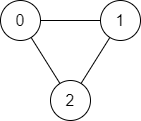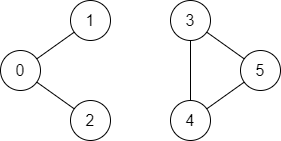# [Leetcode] 1971. Find if Path Exists in Graph

limelimejiwon·2022년 5월 18일
0

## Python으로 공부하는 코딩테스트

목록 보기
59/67## 📄 Description

There is a bi-directional graph with n vertices, where each vertex is labeled from 0 to n - 1 (inclusive). The edges in the graph are represented as a 2D integer array edges, where each edges[i] = [${u_i}$, ${v_i}$] denotes a bi-directional edge between vertex ${u_i}$ and vertex ${v_i}$. Every vertex pair is connected by at most one edge, and no vertex has an edge to itself.

You want to determine if there is a valid path that exists from vertex source to vertex destination.

Given edges and the integers n, source, and destination, return true if there is a valid path from source to destination, or false otherwise.

### Example 1:Input: n = 3, edges = [[0,1],[1,2],[2,0]], source = 0, destination = 2
Output: true
Explanation: There are two paths from vertex 0 to vertex 2:
- 0 → 1 → 2
- 0 → 2

### Example 2:Input: n = 6, edges = [[0,1],[0,2],[3,5],[5,4],[4,3]], source = 0, destination = 5
Output: false
Explanation: There is no path from vertex 0 to vertex 5.

### Constraints:

• $1 <= n <= {2 * 10^5}$
• $0 <= edges.length <= {2 * 10^5}$
• edges[i].length == 2
• $0 <= {u_i}, {v_i} <= n - 1$
• ${u_i} != {v_i}$
• 0 <= source, destination <= n - 1
• There are no duplicate edges.
• There are no self edges.

## 💻 My Submission

class Solution:
def validPath(self, n: int, edges: List[List[int]], source: int, destination: int) -> bool:
graph=defaultdict(list)
# make graph
for n1, n2 in edges:
graph[n1].append(n2)
graph[n2].append(n1)

visited=[]
route=set([source])
while route:
node=route.pop()
if node==destination:
return True
# mark visited node
visited.append(node)
for d in graph[node]:
if d not in visited:
return False

## 🎈 Better Clean Code-DFS

class Solution:
def validPath(self, n: int, edges: List[List[int]], start: int, end: int) -> bool:
neighbors = defaultdict(list)
for n1, n2 in edges:
neighbors[n1].append(n2)
neighbors[n2].append(n1)

def dfs(node, end, seen):
if node == end:
return True
if node in seen:
return False

for n in neighbors[node]:
if dfs(n, end, seen):
return True

return False

seen = set()
return dfs(start, end, seen)

## 🎈 Better Clean Code-BFS

class Solution:
def validPath(self, n: int, edges: List[List[int]], start: int, end: int) -> bool:
neighbors = defaultdict(list)
for n1, n2 in edges:
neighbors[n1].append(n2)
neighbors[n2].append(n1)

q = deque([start])
seen = set([start])
while q:
node = q.popleft()
if node == end:
return True
for n in neighbors[node]:
if n not in seen:
return False